# Proj RSA1: Using Very Small Keys (15 pts.)

What you need:
• Any computer with Python.

## Purpose

To calculate RSA parameters and use them, in a very simple case with small numbers.

## Summary

Here's a diagram from the textbook showing the RSA calculations.## 1. Choose Two Primes: p and q

Let's use p = 7 and q = 23

## 2. Compute n = p * 2

Start Python in interactive mode. On a Mac or Linux box, you can do that by typing this command into a Terminal window:
``` python ```
Execute these commands to store p and q in the Python interpreter, and calculate n
```p = 7 q = 23 n = p * q print p, q, n ```
The parameters print out, as shown below. n = 161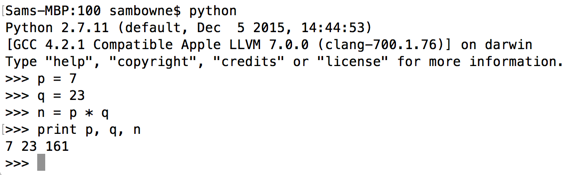## 3. Compute φ(n) = (p-1) * (q-1)

Execute these commands (I don't know how to use Greek in Python, so we'll use "phin" instead of φ(n)):
```phin = (p-1) * (q-1) print p, q, n, phin ```
The parameters print out, as shown below. phin = 132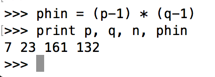## 4. Select Public Exponent e

e has to be between 1 and phin - 1 and it has to not share any divisors with phin. One simple way to meet these requirements is to pick a small prime number.

Let's use e = 5

## 5. Compute Private Key d

We need to find a d with this property:
(d * e) mod phin = 1
First let's set e and perform a simple multiplication.

Execute these commands. As shown below, 20 * 5 is 100, as you'd expect.

```e = 5 print 20 * e ```Now let's use modular arithmetic, with a modulus of phin, that is, 132. In Python, we use a % character to indicate a modulus.

Modulus 132 means there is no number larger than 131. Adding 1 to 131 makes it roll back to 0. The numbers are on a ring, as shown below.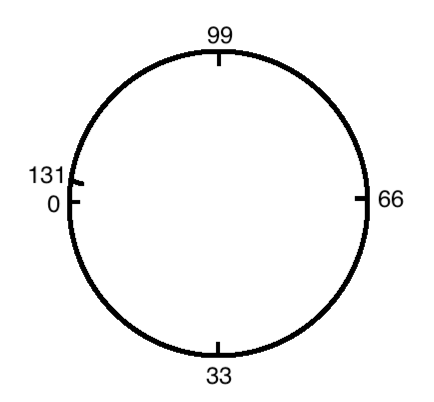Execute these commands. As shown below, 132 modulus phin is zero.

```print 100 % phin print 130 % phin print 131 % phin print 132 % phin ```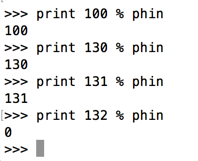Now let's calculate the result for values of d from 20 through 25.

Execute these commands:

```print (20 * e) % phin print (21 * e) % phin print (22 * e) % phin print (23 * e) % phin print (24 * e) % phin print (25 * e) % phin print (26 * e) % phin print (27 * e) % phin ```
We weren't able to find a good value for d -- 26 resulted in 130, and 27 resulted in 3, as shown below. We went right past 1.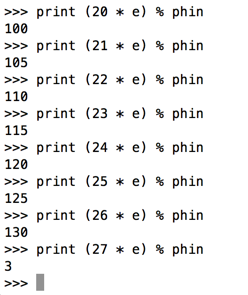We'll have to use larger values and try to hit 1 the next time we go around the ring.

Execute these commands:

```print (50 * e) % phin print (51 * e) % phin print (52 * e) % phin print (53 * e) % phin ```
We have a winner, as shown below! Let's use d = 53## Encrypting a Message

Meghan wants to get private email, so she uses the values above to generate her key pair, as shown below.

She publishes her public key to the cloud.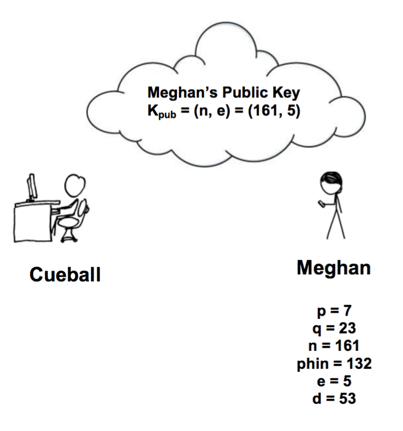Cueball wants to send Meghan this message:

```Hi! ```
We can only send numbers. Let's encode that message with ASCII using the table below.

So the message is:

```72 105 33 ```
A public key contains two numbers: n and e. To encrypt a message x, use this formula:Execute these commands:

```print 72 ** e % n print 105 ** e % n print 33 ** e % n ```
As shown below, the encrypted message is 151 119 157## Decrypting the Message

To decrypt a message, use this formula:Execute these commands:
```d = 53 print 151 ** d % n print 119 ** d % n print 157 ** d % n ```
As shown below, the decrypted message is 72 105 33 or Hi!.## Challenge 1a: Cueball's Keys

Cueball will use these values: p=13 q=41 e=7

Calculate Cueball's public and private keys.

Use the form below to put your name on the WINNERS PAGE.

 Your Name (without spaces): Cueball's Public Key, like this: (161,5)

## Challenge 1b: Message to Cueball

Meghan sends this message to Cueball. Decrypt it.

460, 364, 144, 153, 501, 402, 19, 311, 501, 280, 501, 80, 153, 382, 296, 153, 311

Hint: use the chr() and ord() functions

Use the form below to put your name on the WINNERS PAGE.

 Your Name (without spaces): Decrypted Message:

## Sources

Cracking short RSA keys
Cueball image
Megan image
Cloud image

Posted 3-23-16 by Sam Bowne